# Profitability Index

The profitability index (PI) or PI index is a measure that is used in finance to assess whether a company should pursue a project or not. The profitability index is strongly related to the Net Present Value (NPV), which we discuss on the page on NPV (insert link). On this page, we explain the PI index formula, provide a profitability index example, At the bottom of this page, we implement a profitability index financial calculator using an Excel spreadsheet.

## PI index formula

Let us start with a discussion of the pi index formula. The profitability index equals the present value of a project’s future cash flows divided by the initial cash investment. Algebraically: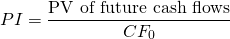An alternative way to formulate the PI index formula is by using the net present value (NPV):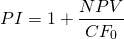This means that if we have the NPV of a project, we can easily calculate the profitability index. We just divide the NPV by the initial cash outlay and add 1.

## PI index decision rule

How should we interpret the I index? If the PI index is larger than one, then we should accept the project. If the PI index is smaller than one, we should not undertake the project.

• if PI > 1, go ahead with the project
• if PI < 1, do not undertake the project

This decision rule is equivalent to using the NPV to choose among projects. In particular, a negative (positive) NPV corresponds with a PI index below (above) 1. Let’s put the above formulae into practice. In the following section we provide a profitability index example.

## Profitability Index example

Let us consider the following profitability index calculation example. Suppose we have two projects with the following initial investment and subsequent cash flows: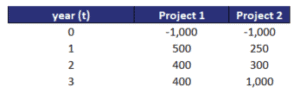The required rate of return is 10%. Now, let us calculate the PI index for both projects: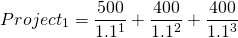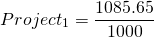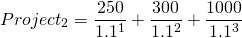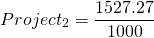Using a required rate of return of 10%, we should opt for project B because it has a profitability index of 1.53, compared to only 1.09 in the case of project A.

## Summary

On this page, we discussed the PI index in finance. The index is used to evaluate whether or not a company should undertake a project. The PI index is a profitability measure that yields results that are identical to the net present value decision rule.